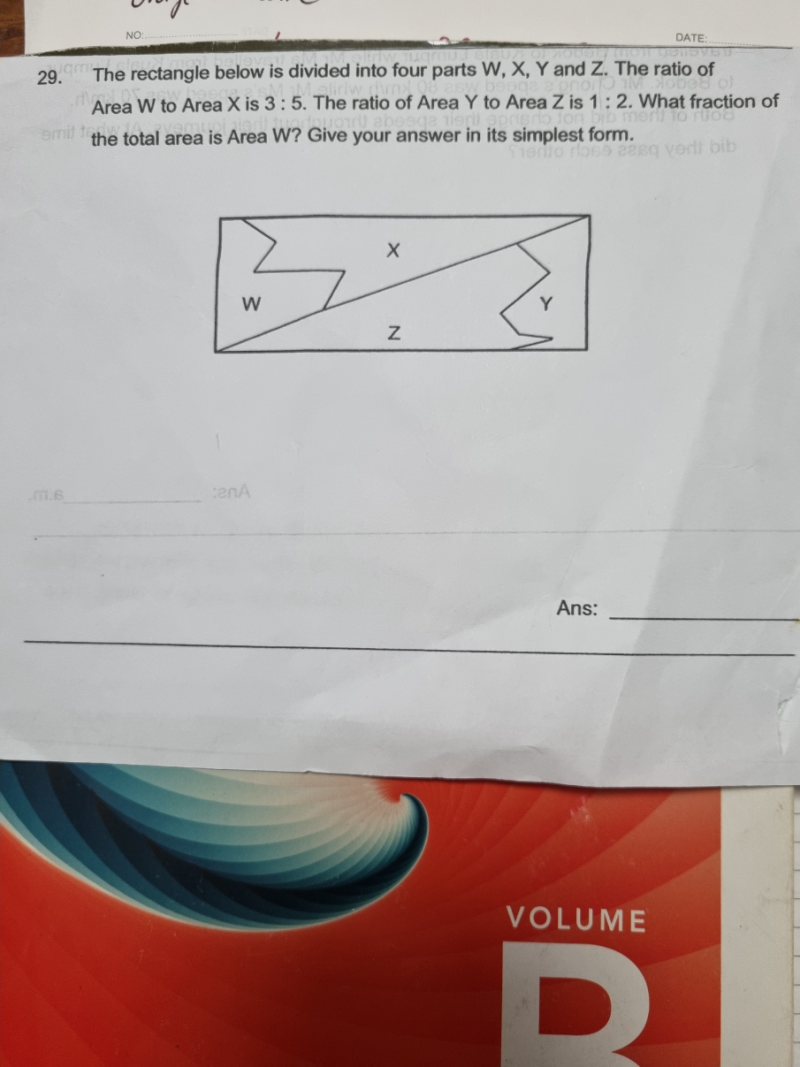# QuestionThank you

Area W               Area   Z                  Area  Y                  Area Z

3/8                     5/8                        1/3                        2/3

In order to be able to compare all their areas, let’s make them have the same denominators

9/24                 15/24                     8/24                      16/24

Since they are all divided into 24 equal parts for both now, the numerators will remain as its original size but the overall rectangle equal parts will become:

The total parts of the rectangle = 24 + 24 = 48

Hence Area W out of total area =  9/48 = 3/16

0 Replies 2 Likes ✔Accepted Answer